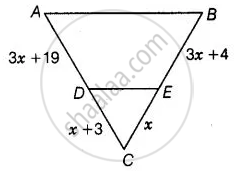# Find the value of x for which DE || AB in figure. - Mathematics

Sum

Find the value of x for which DE || AB in figure.#### Solution

DE || AB

Using basic proportionality theorem,

(CD)/(AD) = (CE)/(BE)

∴ If a line is drawn parallel to one side of a triangle such that it intersects the other sides at distinct points, then, the other two sides are divided in the same ratio.

Hence, we can conclude that, the line drawn is equal to the third side of the triangle.

⇒ (x + 3)/(3x + 19) = x/(3x + 4)

(x + 3) (3x + 4) = x (3x + 19)

3x2 + 4x + 9x + 12 = 3x2 + 19x

19x – 13x = 12

6x = 12

∴ x = 12/6 = 2

Concept: Basic Proportionality Theorem (Thales Theorem)
Is there an error in this question or solution?

#### APPEARS IN

NCERT Mathematics Exemplar Class 10
Chapter 6 Triangles
Exercise 6.3 | Q 2 | Page 67

Share